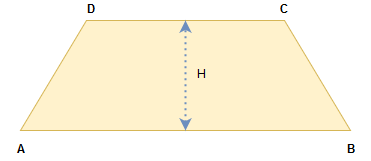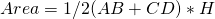Quadrilaterals- a simple closed figure surrounded by four line segment is called a quadrilaterals. It has four sides , four angles, four vertices and two diagonals. The sum of all angles are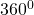.
Adjacent side- having a common end point. e.g PQ & QR
Opposite side- do not have any common point. eg. PQ, RS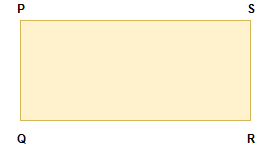Similarly the adjacent angles opposite angle.
The sum of the angle of a quadrilateral is.

Rectangle– a quadrilateral in which opposite side are parallel & equal & each angle is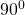called a rectangle.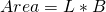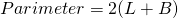Square– a rectangle in which all sides are equal opposite side are, parallel and each angle is, called a square.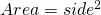Parallelogram– a quadrilateral opposite sides are parallel & opposite angles are equal is called parallelogram.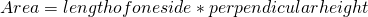or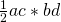(diagonals)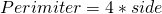Rhombus– a quadrilateral which all side are equal , opposite side are parallel and opposite angles are equal is called rhombus.
Trapezium– a quadrilateral having one opposite side is parallel is called trapezium. When if its non parallel sides are equal is called isosceles trapezium. a quadrilateral is called if their adjacent sides are equal and opposite sides are unequal.

Trapezoid: